Published 25.09.2003. Updated 05.10.2003.

Ph. M. Kanarev,

A.I. Tlishev, D.A. Bebko

E-mail: kanphil@mail.kuban.ru

GENERATORS OF GLOBAL (CLEAN) ENERGY

INTRODUCTION

The global energy problems have been discussed for a long time; they are well known. Depletion of natural energy carriers (oil, gas and coal) is the first problem. Environmental safety of energy carriers is the second problem. It is generally recognized that the solution of the issues connected with the aggregate of these two problems is of a global character. The scientific research results, which should belong to global (clean) energy, originate from it. First and foremost, they are such scientific investigations, which result in the possibility to use an inexhaustible and environmental friendly energy carrier. It has been known for a long time. It is hydrogen produced from water.

Why hydrogen and why from water? Because when hydrogen is burnt, water is formed again; thus, the energy carrier is inexhaustible. As far as inexhaustibility and environmental safety are concerned, hydrogen has no competitors. But the implementation of these qualities is restricted by large energy expenses for hydrogen production from water. For hydrogen production, modern electrolyzers use by 10 or 20% more energy when it is produced while hydrogen is burnt.

It is easy to imagine what financial and intellectual resources of the world are included in the search of the ways how to reduce energy expenses for hydrogen production from water. In Russia, many scientific institutions of branch science and educational institutions are busy with this problem. There exists a research hydrogen institute. The associations of scientists on hydrogen energetics have been established in USA and Europe.

Energy problem is global not due to depletion of oil and gas, but due to their environmental hazard. But the confirmations exist that the world owners of energy resources do not bother about the environmental hazard problem of modern energy carriers.

In history of science, the facts of annihilation of the scientists who have  success in energy expense reduction for hydrogen production from water have already been registered. The orderers of these actions think that they will lose their profits with the rise of hydrogen energetics. They do not understand that this rise cannot be abrupt. It is impossible to substitute hydrogen energetics infrastructure for energy infrastructure of the existing energy carriers during one year or even during ten years. Besides, hydrogen energetics infrastructure will be created not on a blank space. It will be integrated into the existing infrastructure of energetics gradually; and its owners will automatically become the owners of hydrogen energetics. The first step has already been made in this direction. In USA, a decision has been adopted to equip all filling stations with the pumps to fill the cars with hydrogen.

It is known that the theoretical investigation results publication in press is a priority. Usually, such priority is a personal one. Usually, a patent is a priority of the experimental investigation results. As a rule, this patent belongs to a group of authors. A published patent is a jinnee released from a bottle. No finesse of the authors to encumber a reproduction of experimental data given in a patent without the participation of the authors can stop the process of their implementation. Thus, the authors or a group of the authors who have filed an application for a patent are deprived automatically of the opportunity to influence the process of practical realization of their ideas.

It is known that if it becomes possible to reduce energy expenses for hydrogen production of water 5fold, it will be the cheapest energy carrier. Russia has already got technology, which reduces these expenses 10fold and more. But another direction is more perspective. What is the use to break down water into hydrogen and oxygen and to use hydrogen as fuel for heating, for example, water in heating systems? Is it possible to make water generate heat? As it happens, it is possible.

In Russia, three firms (Yusmar, Termovikhr and Noteka) sell cavitation heating equipment with energy performance index up to 150%. Official science looks awry at this activity, because such results conflict with one of the main laws of physics: law of conservation of energy. But market profit is stronger than this law.

Engineering practice has already proved that additional energy in the form of heat is generated in the ventilation systems and in the water cavitation systems. Deep scientific analysis of this problem shows that physical vacuum is the most probable source of additional energy in the systems of ventilation and water cavitation. Valence electrons of destroyed molecules of water take energy from physical vacuum and release it during repeated fusion of these molecules.

Why is additional energy generated in the air systems of ventilation and in water cavitation systems? Because they are mechanical systems; mechanical destruction of chemical links requires half energy as compared with thermal destruction of these links. This is the main reason why one fails to increase energy performance index of cavitation processes over 200%.

An increase of efficacy of any process by 30 or 50% is a good result; if it is obtained, it is possible to get even better result. What if a water molecule is destroyed not mechanically, but electrodynamically? In this case, it becomes possible to find resonance frequencies of impact on the molecules and to reduce considerably electrical energy expenses for their destruction. Subsequent fusion of destroyed molecules will release prescribed quantity of energy unavoidably. It is a simple idea, and it has already been implemented (Tabl. 4-6).

In order to get results given below, it is necessary to get new microworld physchemistry knowledge, which has already been published. Every month more than 1000 foreign scientists get acquainted with this knowledge visiting the site   http://Kanarev.innoplaza.net

The Russian speaking readers get this information on the sites  http://www.ikar.udm.ru/sb28-2.htm  and  http://www.n-t.org/tp/ts/eb.htm

# LOW CURRENT PROCESS OF WATER ELECTROLYSIS

Low voltage process of water electrolysis is known from Faraday’s times. It is widely used in modern industry. Voltage of 1.6-2.3 volts is operation voltage between the anode and the cathode of the electrolyzer; current strength is tens and hundreds of amperes. In accordance with Faraday’s law, energy consumption for production of one cubic meter of hydrogen is nearly 4 kWh  in this case.

The analysis of the water molecule structure (Fig. 1) worked out by us shows the possibility of water electrolysis at minimal current and even without it. The protons of the hydrogen atoms in water molecules can be combined with each other and can form clusters. As a result, an orthohydrogen molecule is formed (Fig. 2). A question arises: is it possible to separate this molecule from such cluster? The results of answers on this question are given in Tables 1, 2 and 3.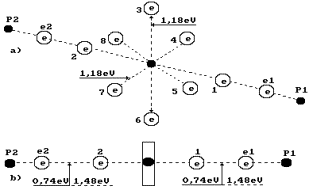Fig. 1. Water molecule diagram: 1,2,3,4,5,6,7,8 are numbers of the electrons of the oxygen atom; P1, P2 are the hydrogen atom nuclei (the protons); e1 and e2 are the electron numbers of the hydrogen atoms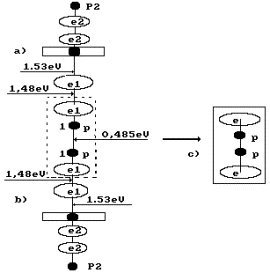Fig. 2. Formation diagram of  orthohydrogen: a) and b) water molecule diagrams; c) orthohydrogen

It is known that a gram-atom is equal to atomic mass of substance; a grammolecule is equal to molecular mass of substance. For example, the grammolecule of hydrogen in the water molecule is equal to two grams; the gram-atom of the oxygen atom is 16 grams. The grammolecule of water is equal to 18 grams. Hydrogen mass in a water molecule is 2x100/18=11.11%; oxygen mass is 16x100/18=88.89%; this ratio of hydrogen and oxygen is in one liter of water. It means that 111.11 grams of hydrogen and 888.89 grams of oxygen are in 1000 grams of water.

One liter of hydrogen weighs 0.09 g; one liter of oxygen weighs 1.47 g. It means that it is possible to produce 111.11/0.09=1234.44 liters of hydrogen and 888.89/1.47=604.69 liters of oxygen from one liter of water. It appears from this that one gram of water contains 1.23 liters of hydrogen. Energy consumption for production of 1000 liters of hydrogen is 4 kWh and for one liter 4 Wh. As it is possible to produce 1.234 liters of hydrogen from one gram of water, 1.234x4=4.94 Wh is spent for hydrogen production from one gram of water now.

Instruments and equipment used during the experiment

Special experimental low current electrolyzer (Fig. 3); voltmeter of the highest accuracy class (accuracy class of 0.2 GOST 9711-78); ammeter of the highest accuracy class (accuracy class of 0.2 GOST 9711-78)’ electronic scale with scale division value of 0.1 and 0.01 g; stop watch with scale division value of 0.1 s.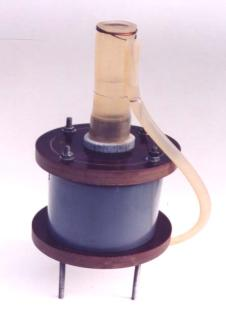Fig. 3. Low current electrolyzer in the closed form (in the process of patenting)

Table 1

 Indices Sum 1 - duration of the experiment  t, h 6.000 2 – readings of voltmeter V, volts 3.750 3 – ammeter readings I, amperes 0.020 4 – power P, watts hour (P=VxIxτ/60) 0.450 5 – continue of experiment without input energy in 6 series, min 0.000 6 – mass difference, grams 0.52 7 – mass of evaporated water, grams 0.01x6=0.06 8 – mass of water converted in hydrogen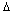m, grams 0.46 9 – specific power P’=P/m, Watt/gram of water 0.98 10 – existing  specific power P’’, Watt/gram of water 4.94 11 – the reducing  power on the production of hydrogen, times K=P’’/P’ 5.04 12– quantity of released hydrogen, ΔМ =0.46x1.23x0.09=0.051, grams 0.051 13 – energy content of hydrogen being obtained      (Е=0.051х142/3,6)=2.008 Wth 2.008 14- energy efficacy of low ampere process of water electrolysis (Eх100/P), % 446.2

Table 2

 Indices Sum 1 - duration of the experiment with input energy in 6 series t, min 6x30=180.0 2 – readings of voltmeter V, volts 3.750 3 – ammeter readings I, amperes 0.022 4 – power P, watts hour (P=VxIxτ/60) 0.247 5 – continue of experiment without input energy in 6 series, min 6x30=180.0 6 – mass difference, grams 0.45 7 – mass of evaporated water, grams 0.1x6=0.06 8 – mass of water converted in hydrogenm, grams 0.39 9 – specific power P’=P/m, Watt/gram of water 0.63 10 – existing  specific power P’’, Watt/gram of water 4.94 11 – the reducing  power on the production of hydrogen, times K=P’’/P’ 8.40 12– quantity of released hydrogen, ΔМ =0.39x1.23x0.09=0.043, grams 0.043 13 – energy content of hydrogen being obtained      (Е=0.043х142/3,6)=1.70 Wth 1.70 14- energy efficacy of low ampere process of water electrolysis (Eх100/P), % 689.0

Table 3

 Indices Sum 1 - duration of the experiment with input energy in 6 series t, min 6x5=30 2 – readings of voltmeter V, volts 13.60 3 – ammeter readings I, amperes 0.020 4 – power P, watts hour (P=VxIxτ/60) 0.136 5 – continue of experiment without input energy in 6 series, min 6x55=330 6 – mass difference, grams 0.44 7 – mass of evaporated water, grams 0.01x6=0.06 8 – mass of water converted in hydrogenm, grams 0.38 9 – specific power P’=P/m, Watt/gram of water 0.358 10 – existing  specific power P’’, Watt/gram of water 4.94 11 – the reducing  power on the production of hydrogen, times K=P’’/P’ 13.80 12– quantity of released hydrogen, ΔМ =0.38x1.23x0.09=0.042, grams 0.042 13 – energy content of hydrogen being obtained      (Е=0.042х142/3,6)=1.66 Wth 1.66 14- energy efficacy of low ampere process of water electrolysis (Eх100/P), % 1220.0

Note: In Tables 1, 3, the results of the experiment are given when frequency of nearly 500 Hz has been generated in the power supply, in table 2 – without frequency.

First of all, we should note that the anode and the cathode are made of one and the same material: steel. It excludes the possibility of formation of a galvanic cell. If we analyze Tables 1, 2 and 3, we’ll see the electrolysis process takes place at very low current of 0.02 A; that’s why it has been called low current one. Further, this process consisted of two cycles in some experiments; in one cycle, the electrolyzer is connected to the power line; in another cycle, it is disconnected (Tables 2, 3).

Gas generation process is manifested by release of the bubbles being formed. The bubbles go on being released after the electrolyzer is disconnected from the supply line (Tables 2 and 3). When the electrolyzer is de-energized, gas release intensity is reduced, but it is not stopped during many hours. It is proved by the fact that electrolysis takes place at the expense of potential difference on the electrodes.

After electrolyzer de-energizing, gas release during a long period of time proves the fact that the molecules of oxygen and hydrogen are formed without the electrons emitted by the cathode, i.e. at the expense of the electrons of the water molecule itself.

Simplicity and 100% reproducibility of the experiments being described afford ground for the fact that mankind has got a chance to avoid energy famine and environmental crisis.

WATER ELECTRIC GENERATOR OF HEAT

We have already shown that energy of physical vacuum taken by valence electrons of the molecules after their mechanical destruction and emitted by these electrons within the repeated fusion of the molecules is the most probable source of additional energy generated by the ventilation systems and the cavitation ones. It is explained by the fact that half as much energy is spent for mechanical destruction of the molecules than for thermal destruction of these molecules. Valence electrons of the molecules being destroyed mechanically absorb energy from physical vacuum in order to restore their energy indices and emit it during the repeated fusion of these molecules.

As half as much energy is spent for mechanical destruction of the molecules than for thermal one, energy effectiveness index of such processes cannot exceed two. But if this hypothesis is correct, there is a possibility to increase energy effectiveness index of this process considerably when the molecules are destroyed electrodynamically. In this case, there is a possibility to find resonance modes of electrodynamic destruction of the molecules and to reduce energy consumption for this process considerably. Further fusion of the molecules being destroyed electrodynamically will release required quantity of energy, which will exceed considerably the energy being spent.

EXPERIMENTAL PART

The main task of the experiment was to check the hypothesis: "Electrodynamic influence on the water molecules gives the possibility to reduce energy expenses on destruction of their chemical bonds significantly; further fusion of these molecules increases considerably the output of additional energy in the form of heat".

In order to solve this task, special experiments were carried out connected with electrodynamic destruction of chemical bonds of water molecules with electric pulses of various frequencies. The diagram of the installation used for experimental investigations is shown in Fig. 4; the photo of the experimental generator of heat is shown in Fig. 5.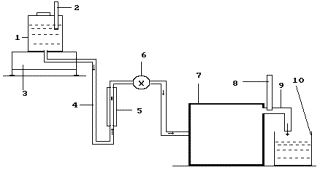Fig. 4. Diagram of the experimental installation: 1 - reservoir for solution; 2- thermometer; 3- electronic scales; 4 - solution feed duct; 5- rotameter; 6- solution feed regulator; 7-a special thin plasma generator is in the process of patenting; 8 - thermometer; 9- heated solution discharge.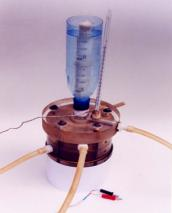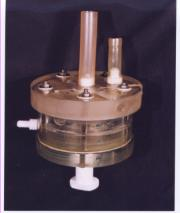Fig. 5. Photos of heat generator

The results of the experiments are given in Figs  6-9 and in Tables 4 -5.

The oscillogram of voltage pulses is given in Fig.  6; the oscillogram of current pulses influencing the generator of heat in one of the experiments carried out with pulse frequency of nearly 100 Hz is given in Fig. 7. As it is clear from the oscillograms, the pulses of both voltage and current have an exponential form being close to the triangular one with a sharp edge and a shallow declination. The design duty factor for these pulses is Z » 0.039. Mean amplitude of voltage pulses is equal to power supply voltage of the pulse generator: 250 V. Thus, a mean component of voltage pulses being brought to the generator of heat is equal to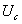= 0.039 х 250 = 9.75 V. In this experiment, voltmeter readings were 10.0 V.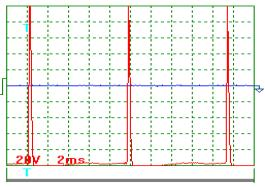Fig. 6.  Oscillogram of power supply voltage pulses at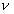» 100 Hz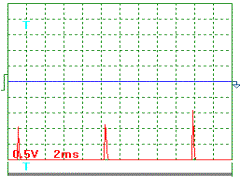Fig. 7.  Oscillogram of power supply current pulses at» 100 Hz

A current pulse oscillogram in this experiment is shown in Fig. 7. Current was measured as voltage drop at a measuring resistor with resistance of  0.1 ohm included into the supply circuit of generator of heat. As it is clear, mean amplitude of current pulses is 1.3 / 0.1 = 13 А, and mean component value is equal to: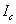= 0.039 х 13 =  0.51 А. During measurements, the ammeter showed current of   0.50 A.

On the basis of the oscillographic measurement data, mean value of electric power has proved to be Р = 9.8х0.51 = 5.0 W. The experiment lasted for 300 seconds. Thus, electric energy of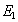=5.0 х 300 = 1500 J = 1.5 kJ entered the generator of heat. During this period, it heated 0.55 kg of solution by 12 degrees. Energy value of this heat was equal to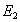=4.19х0.55х12=27.65 kJ. Efficiency index of energy process was  К =  Е2 / Е1 = 27.65 / 1.5 = 18.43, or 1843%.  It corresponds (with accuracy of up to 5% being characteristic of oscillographic check) to energy efficiency index being determined with the help of the voltmeter and the ammeter (s. Table 4).

Table 4

Experimental indices of the water electric generator of heat  with electric pulse frequency of nearly 100 Hz

 Indices Mean 1. Mass of the solution, which has passed through the generator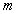,  kg. 0.55 2. Temperature of solution at the input of the generator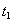, degrees 26.00 3. Temperature of the solution at the output of the generator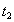, degrees 38.00 4. Temperature difference of the solution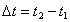, degrees 12.00 5. Durability of the experiment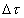, s 300.00 6. Reading of voltmeter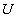, V 10.50 6’. Reading of oscillograph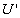, V 9.75 7. Reading of ammeter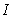, A 0.50 7’. Reading of oscillograph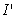, A 0.51 8. Electric power consumption,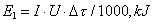1.50 9 – power spent for heating of the solution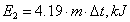27.65 10 – reactor efficiency index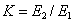18.43

In Fig. 8, the oscillogram of voltage pulses is given. In Fig. 9, the oscillogram of current pulses being registered during another experiment with pulse frequency of nearly 300 Hz is given. According to these oscillograms, the duty factor calculation has given the result of Z = 0.11. With mean values of amplitudes of pulses of voltage and current being equal to 250 V and 10.6 A, respectively, the mean components of voltage and current arriving into the generator of heat have been:= 0,11 х 250 = 27.5 V;= 0.11 х 10.6 = 1.17 A. According to the readings of the voltmeter and the ammeter, mean values of voltage and current were 25.0 V and 1.25 A in this experiment. In this connection, mean value of electric power supplied to the generator of heat was 27.5 х 1.17 = 32.18 W according to the data of the oscillographic measurements and 25 х 1.25 = 31.25 W according to the data of the pointer indicators. Divergence in this methods of mean power determination did not exceed 5% as well.

The energy efficiency calculation results of the generators of the heat for both methods of measurement with pulse frequency of nearly 300 Hz are given in Table 5. They are close in their values as well.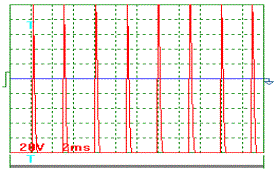Fig. 8. Oscillogram of supply voltage pulses at» 300 Hz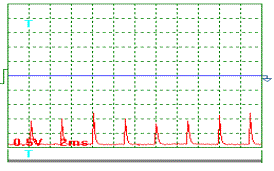Fig. 9. Oscillogram of supply current  pulses at» 300 Hz

Table 5

Experimental indices of the water electric generator of heat  with electric pulse frequency of nearly 300 Hz

 Indices Mean 1. Mass of the solution, which has passed through the generator,  kg. 0.41 2. Temperature of solution at the input of the generator, degrees 26.00 3. Temperature of the solution at the output of the generator, degrees 76.00 4. Temperature difference of the solution, degrees 50.00 5. Durability of the experiment, s 300.00 6. Reading of voltmeter, V 25.00 6’. Reading of oscillograph, V 27.5 7. Reading of ammeter, A 1.25 7’. Reading of oscillograph, A 1.17 8. Electric power consumption,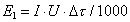, kJ 9.38 9. Power spent for heating of the solution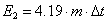, kJ 85.90 10. Generator  efficiency index9.16

PROTOCOL  OF CONTROL TEST

Table 6

Supply voltage and current were measured with the help of a voltmeter, an ammeter and an oscillograph

(Fig. 10-13)

 Indices 1 2 3 Mean 1 – mass of the solution, which has passed through the reactor m,  kg. 0.47 0.432 0.448 0.450 2 – temperature of solution at the input of the reactor  t1, degrees 22 22 22 22 3 – temperature of the solution at the output of the reactor t2, degrees 66 66 65 65.67 4 – temperature difference of the solution Dt= t2 - t1, degrees 44 44 43 43.67 5 – durability of the experiment  Dt, s 300 300 300 300 6 – reading of voltmeter V, V 4.5 4.5 4.5 4.50 6’. Reading of oscillograph, V 4.47 4.47 4.47 4.47 7 – reading of ammeter I, A 2.1 2.1 2.1 2.1 7’. Reading of oscillograph, A 2.2 2.2 2.2 2.2 8 – electric power consumption according to indices of voltmeter and ammeters, E2=I×V×Dt, kJ 2.84 2.84 2.84 2.84 9 – power spent for heating of the solution, E3=4.19×m×Dt, kJ 79.64 80.01 80.72 80.46 10 – reactor efficiency index K= E3/ E2 28.04 28.17 28.42 28.21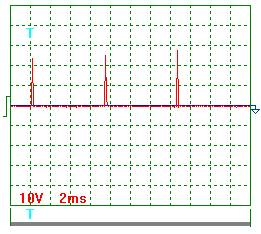Fig. 10. Tension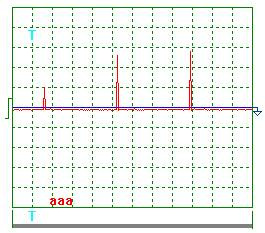Fig. 11. Tension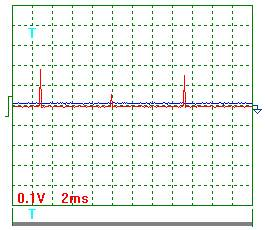Fig. 12. Current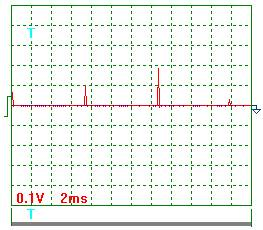Fig. 13. Current

Process parameter calculation according to the oscillograms (Fig. 10-13) to the check test protocol (Table 6) gave the following results.

Pulse scale 10.

Mean voltage amplitude according to Fig.10 and Fig. 11:

Ua = (23+25+28+10+26+29) х 10/6 = 235 V.

Mean current amplitude according to Fig. 12 and Fig. 13:

Iа = (20+6+17+7+10+19+3) х 10/7 = 117 A.

Pulse repetition period T = 7.4 ms.

Pulse duration t = 0.28 ms.

Pulse frequency f = 1000/7.4 = 135.1 Hz.

Relative pulse duration S = 7.4/0.28 = 26.32.

Space factor Z = 0.5/26.32 = 0.019.

Mean value of pulse voltage импульсов Um = 0.019 х 235 = 4.47 V.

Mean value of current in pulses Im = 0.019 х 117 = 2.22 A.

Thus, it is possible to consider that an experimental check of energy efficiency of the water electric generator of heat with the help of two methods gives practically the same results and confirms the above-mentioned hypothesis concerning the possibility of additional energy production in the processes being considered. It can be noted that as during measurements the pointer instruments of high class of accuracy of 0.2 have been used (relative conventional gauging error does not exceed 0.2%) and oscillographic measurement accuracy is much lower (usually nearly 5%), the readings of the voltmeter and the ammeter should be considered as more accurate ones.

Commercial efficiency of the water electric generator of heat will depend on pulse generator economies. As efficiency of powerful pulse generators can be near unit, energy efficiency should not differ greatly from the data being obtained during laboratory investigations for the industrial-scale plants with the use of the generators of heat being considered.

The analysis of energy balance of the molecules with covalent bonds shows the possibility of formation of additional thermal energy with the energy efficiency index, which exceeds unit greatly, and the experiments confirm this hypothesis earnestly.

Simplicity and hundred per cent reproducibility of the experiments being described open a prospect for quick commercialization of the water electric generator of heat.

CONCLUSION

Thus, the convincing theoretical and experimental proofs of existence of a method, which reduces energy consumption for hydrogen production from water 10folds and more, have been got.

The method of conversion of electric energy into thermal energy with energy efficiency index of more than 2000% has been found.

The way of a transfer to economical and environmental friendly power engineering is opened. But it will not be an easy one. There will be a lot of work concerning optimization of the parameters of the global energy generators.

REFERENCES

1. Kanarev Ph.M. The Foundation of  Physchemistry of  Microworld (the second edition). (In Russian)  http://www.ikar.udm.ru/sb28-2.htm

2. Kanarev Ph.M. The Foundation of  Physchemistry of  Microworld. The second edition. (In English). http://book.physchemistry.innoplaza.net

3. Kanarev Ph.M., G.P. Perekoty, D.A. Bebko, A.A. Chernyavsky.  Water Electric Generator of Heat. http://kanarev.heatgenerator.innoplaza.net

4. Kanarev Ph.M. Energy Balance of Fusion Process of Oxygen, Hydrogen and Water Molecules. New Energy Technologies. 2003, Issue No. 3 (12), pages 58-62. (In English).

5. Kanarev Ph.M. Global Energy. New Energy Technologies. 2003, Issue No. 3 (12), 2003, pages 56-57. (In English).

6. Kanarev Ph.M. The Foundation of  Physchemistry of  Micro World. The second edition. (In English). http://book.physchemistry.innoplaza.net

7. Kanarev Ph.M. Energy Balance of Fusion Process of Oxygen, Hydrogen and Water Molecules. New Energy Technologeis. 2003, Issue Nr 3 (12).

9. Ph.M. Kanarev, V.V. Podobedov. Device for production of thermal energy and steam-and-gas mixture.

Patent No.  2157862.

10. Ph.M. Kanarev, E.D. Zykov, V.V. Podobedov. Device for production of thermal energy, hydrogen and oxygen. Patent No.   2157861.

11. Ph.M. Kanarev, V.V. Konarev, V.V. Podobedov, A.B. Garmashov.  Device for production of thermal energy, hydrogen and oxygen. Patent No.  2175027.

12. Ph.M. Kanarev, V.V. Konarev, V.V. Podobedov. Device for production of thermal energy, hydrogen and oxygen. Patent No.  2167958.

13. Ph.M. Kanarev, V.V. Konarev, V.V. Podobedov. Device for production of thermal energy, hydrogen and oxygen. Patent No.  2167958.

14. Ph.M. Kanarev., Ya.A. Peysakhovich, V.V. Podobedov.  Device for production of thermal energy, hydrogen and oxygen. Patent No.  2177512.

15. Ph.M. Kanarev, V.V. Podobedov, D.V. Korneev, A.I. Tlishev, D.A. Bebko. Device for gas mixture production and transmutation of the atomic nuclei of chemical elements.  Patent No. 2210630.

Webmaster: j_hartikka@hotmail.com

Generators of Global (Clean) Energy by Prof. Kanarev:

http://Kanarev.energygenerators.innoplaza.net

<< Kanarev´s Page